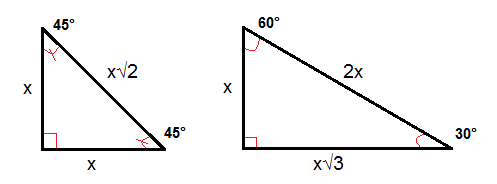# The converse of the Pythagorean theorem and special triangles

If we know the sides of a triangle - we can always use the Pythagorean Theorem backwards in order to determine if we have a right triangle, this is called the converse of the Pythagorean Theorem.

$If\; a^{2}+b^{2}=c^{2}\; then\; \triangle ABC\; is\;a\;right\;triangle.$

When working with the Pythagorean theorem we will sometimes encounter whole specific numbers that always satisfy our equation - these are called a Pythagorean triple. One common Pythagorean triple is the 3-4-5 triangle where the sides are 3, 4 and 5 units long.

There are some special right triangles that are good to know, the 45°-45°-90° triangle has always a hypotenuse √2 times the length of a leg. In a 30°-60°-90° triangle the length of the hypotenuse is always twice the length of the shorter leg and the length of the longer leg is always √3 times the length of the shorter leg.## Video lesson

Find the value of x in the right triangle.